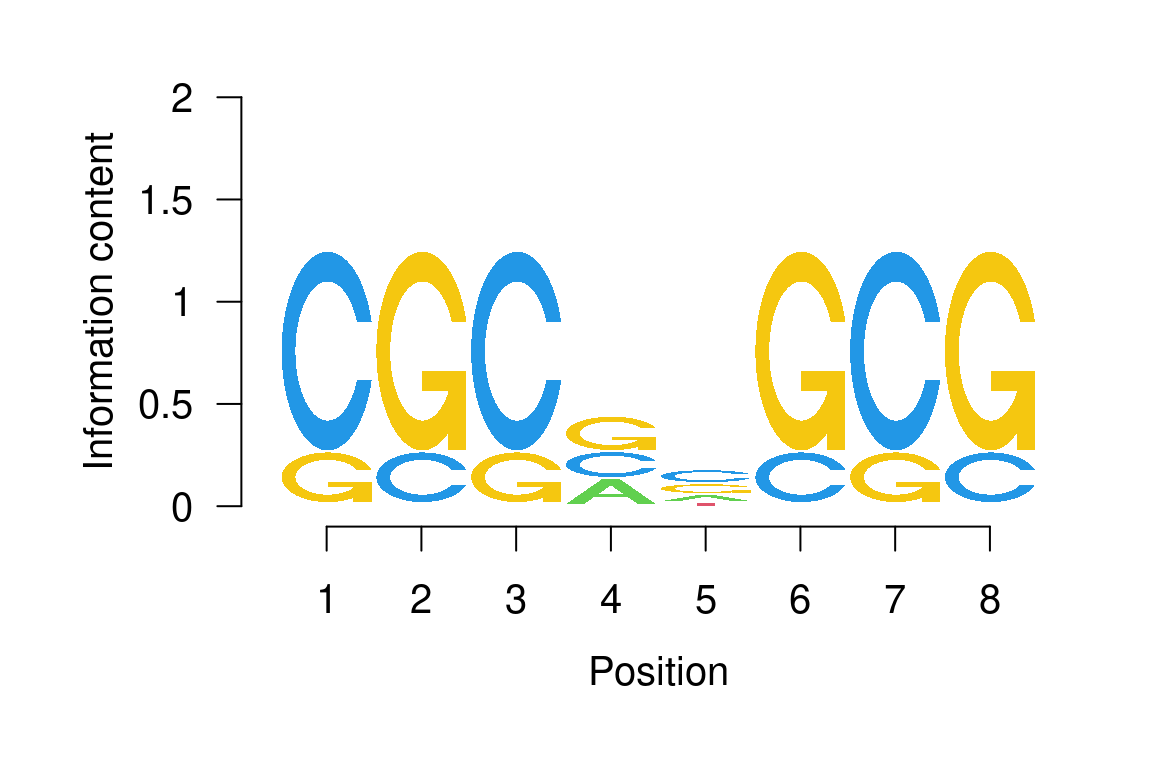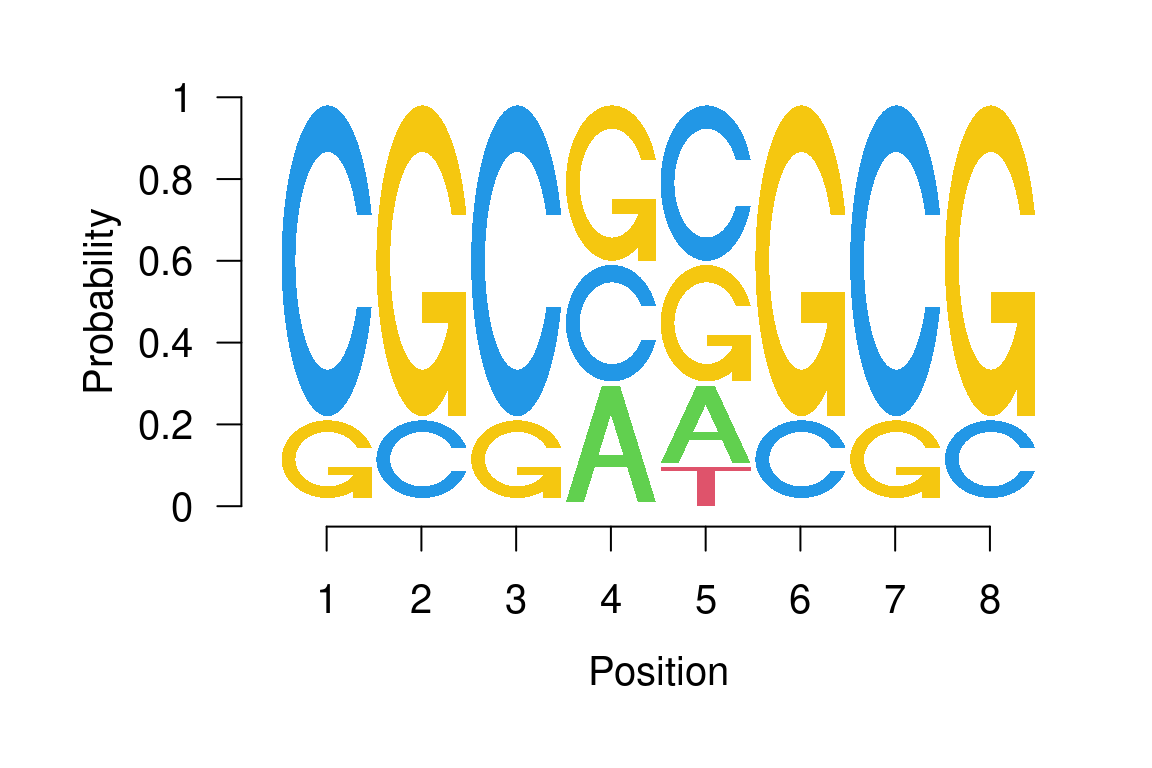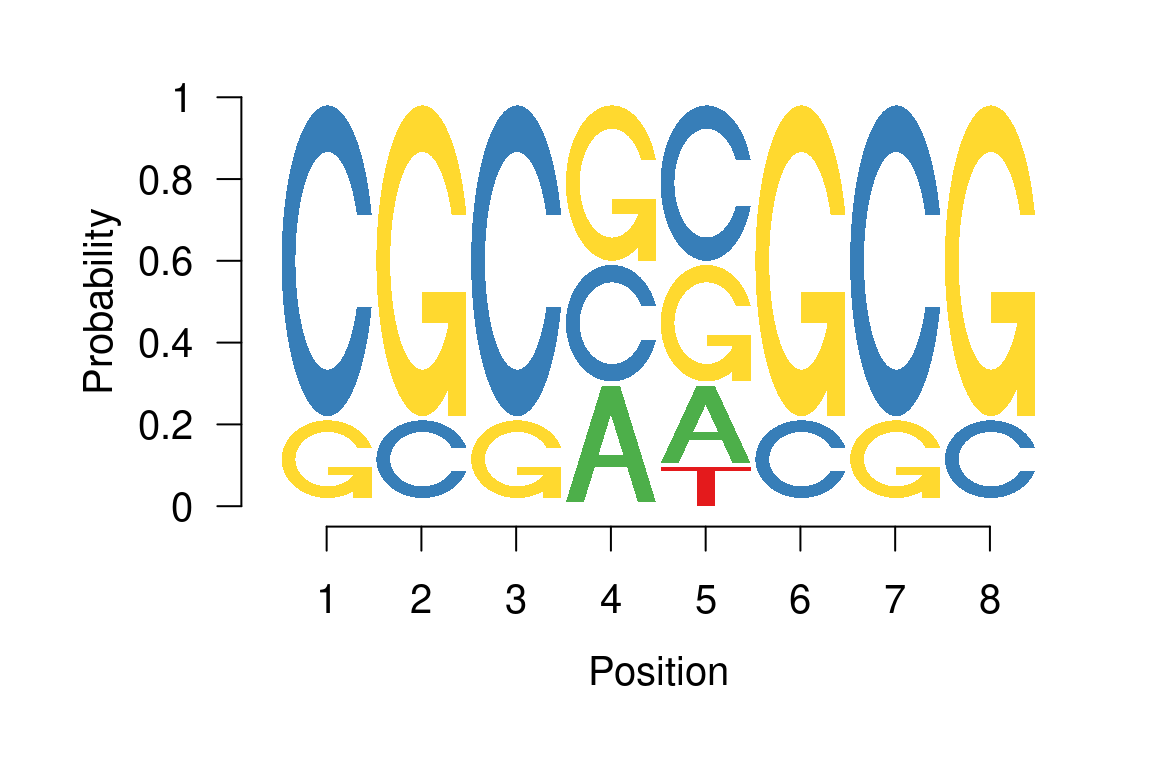Last edited: 08 May 2020

# 1 Introduction

An alignment of DNA or amino acid sequences is commonly represented in the form of a position weight matrix (PWM), a $$J \times W$$ matrix in which position $$(j,w)$$ gives the probability of observing nucleotide $$j$$ in position $$w$$ of an alignment of length $$W$$. Here $$J$$ denotes the number of letters in the alphabet from which the sequences were derived. An important summary measure of a given position weight matrix is its information content profile (Schneider et al. 1986). The information content at position $$w$$ of the motif is given by

$IC(w) = \log_2(J) + \sum_{j=1}^J p_{wj}\log_2(p_{wj}) = \log_2(J) - entropy(w).$

The information content is measured in bits and, in the case of DNA sequences, ranges from 0 to 2 bits. A position in the motif at which all nucleotides occur with equal probability has an information content of 0 bits, while a position at which only a single nucleotide can occur has an information content of 2 bits. The information content at a given position can therefore be thought of as giving a measure of the tolerance for substitutions in that position: Positions that are highly conserved and thus have a low tolerance for substitutions correspond to high information content, while positions with a high tolerance for substitutions correspond to low information content.

Sequence logos are a graphical representation of sequence alignments developed by (Schneider and Stephens 1990). Each logo consists of stacks of symbols, one stack for each position in the sequence. The overall height of the stack is proportional to the information content at that position, while the height of symbols within the stack indicates the relative frequency of each amino or nucleic acid at that position. In general, a sequence logo provides a richer and more precise description of, for example, a binding site, than would a consensus sequence.

# 2 Software implementation

The seqLogo package provides an R implementation for plotting such sequence logos for alignments consisting of DNA sequences. Before being able to access this functionality, the user is required to load the package using the library() command:

library(seqLogo) 

## 2.1 The pwm-class

The seqLogo package defines the class pwm which can be used to represent position weight matrices. An instance of this class can be constructed from a simple matrix or a data frame using the function makePWM():

mFile <- system.file("extdata/pwm1", package="seqLogo")
m
##    V1  V2  V3  V4  V5  V6  V7  V8
## 1 0.0 0.0 0.0 0.3 0.2 0.0 0.0 0.0
## 2 0.8 0.2 0.8 0.3 0.4 0.2 0.8 0.2
## 3 0.2 0.8 0.2 0.4 0.3 0.8 0.2 0.8
## 4 0.0 0.0 0.0 0.0 0.1 0.0 0.0 0.0
p <- makePWM(m)

makePWM() checks that all column probabilities add up to 1.0 and also obtains the information content profile and consensus sequence for the position weight matrix. These can then be accessed through the corresponding slots of the created object:

slotNames(p)
##  "pwm"       "width"     "ic"        "alphabet"  "consensus"
pwm(p)
##     1   2   3   4   5   6   7   8
## A 0.0 0.0 0.0 0.3 0.2 0.0 0.0 0.0
## C 0.8 0.2 0.8 0.3 0.4 0.2 0.8 0.2
## G 0.2 0.8 0.2 0.4 0.3 0.8 0.2 0.8
## T 0.0 0.0 0.0 0.0 0.1 0.0 0.0 0.0
ic(p)
##  1.2780719 1.2780719 1.2780719 0.4290494 0.1535607 1.2780719 1.2780719
##  1.2780719
consensus(p)
##  "CGCGCGCG"

## 2.2 Plotting sequence logos

The seqLogo() function plots sequence logos.

### 2.2.1 Input

1. The position weight matrix for which the sequence logo is to be plotted, pwm. This may be either an instance of class pwm, as defined by the package seqLogo, a matrix, or a data.frame.

2. A logical ic.scale indicating whether the height of each column is to be proportional to its information content, as originally proposed by (Schneider et al. 1986). If ic.scale=FALSE, all columns have the same height.

### 2.2.2 Example

The call seqLogo(p) produces the sequence logo shown in figure ??. Alternatively, we can use seqLogo(p, ic.scale=FALSE) to obtain the sequence logo shown in figure ?? in which all columns have the same height.

seqLogo(p)Figure 1: Sequence logo with column heights proportional to information content

seqLogo(p, ic.scale=FALSE)Figure 2: Sequence logo with uniform column heights

It is also possible to change the default colors by providing a named character vector as a fill argument seqLogo function.

seqLogo(p, fill=c(A="#4daf4a", C="#377eb8", G="#ffd92f", T="#e41a1c"),
ic.scale=FALSE)Figure 3: Sequence logo with user specified colors

The RNA logos are supported as well. In this particular case, the seqLogo will either accept fill colors specified for c("A", "C", "G", "U") letters or c("A", "C", "G", "T") and uses the color specified in element “T” for letter “U”.

r <- makePWM(m, alphabet="RNA")
seqLogo(r, ic.scale=FALSE)